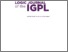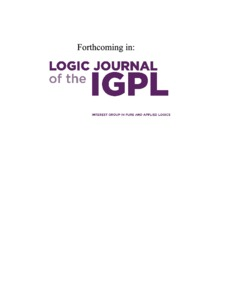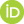# The Quantum Logic of Direct-Sum Decompositions: The Dual to the Quantum Logic of Subspaces

Ellerman, David (2017) The Quantum Logic of Direct-Sum Decompositions: The Dual to the Quantum Logic of Subspaces. [Preprint]Preview
Text
QPL3wCover.pdf

## Abstract

ince the pioneering work of Birkhoff and von Neumann, quantum logic has been interpreted as the logic of (closed) subspaces of a Hilbert space. There is a progression from the usual Boolean logic of subsets to the "quantum logic" of subspaces of a general vector space--which is then specialized to the closed subspaces of a Hilbert space. But there is a "dual" progression. The set notion of a partition (or quotient set or equivalence relation) is dual (in a category-theoretic sense) to the notion of a subset. Hence the Boolean logic of subsets has a dual logic of partitions. Then the dual progression is from that logic of set partitions to the quantum logic of direct-sum decompositions (i.e., the vector space version of a set partition) of a general vector space--which can then be specialized to the direct-sum decompositions of a Hilbert space. This allows the quantum logic of direct-sum decompositions to express measurement by any self-adjoint operators. The quantum logic of direct-sum decompositions is dual to the usual quantum logic of subspaces in the same sense that the logic of partitions is dual to the usual Boolean logic of subsets.

 Export/Citation: EndNote | BibTeX | Dublin Core | ASCII/Text Citation (Chicago) | HTML Citation | OpenURL
 Social Networking:

Item Type: Preprint
Creators:
CreatorsEmailORCIDEllerman, Daviddavid@ellerman.org
Keywords: quantum logic of subspaces, dual quantum logic of direct-sum decompositions
Subjects: Specific Sciences > Mathematics > Logic
Specific Sciences > Physics > Quantum Mechanics
Depositing User: David Ellerman
Date Deposited: 17 Jul 2017 15:55
Item ID: 13212
Subjects: Specific Sciences > Mathematics > Logic
Specific Sciences > Physics > Quantum Mechanics
Date: 2017
URI: http://philsci-archive.pitt.edu/id/eprint/13212

### Actions (login required)View Item